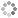# PROWAREtecharticles » current » javascript » three-js » bouncing-ball

## ThreeJS: Bouncing Ball

Two ways to create a bouncing ball using THREE.js; use sine/cosine or basic physics to bounce a ball.

First, the simplest method using sine/cosine, which oscillates between the values -1 and 1:

``````
// NOTE: this is used to modify the y position of a 3D object or group of 3D objects
// NOTE: can use cosine with practically the same results
var bouncePosition = function(bounceSpeed, bounceDistance, height) {
return Math.sin(Date.now() * (bounceSpeed / 100)) * bounceDistance + height;
};
``````

A complete working example:

``````
<!DOCTYPE html>
<html lang="en">
<meta charset="UTF-8">
<meta http-equiv="X-UA-Compatible" content="IE=edge">
<meta name="viewport" content="width=device-width, initial-scale=1.0">
<title>Bouncing Ball</title>
<style>
body {
background-color: white;
}
canvas {
display: block;
width: 300px;
height: 300px;
}
</style>
<body>
<canvas></canvas>
<script src="/js/three.min.js"></script>
<script type="text/javascript">

(function () {

var canvas = document.getElementsByTagName("canvas");

// NOTE: create the scene to place objects in
var scene = new THREE.Scene();
scene.background = new THREE.Color(0xFFFFFF);
scene.matrixWorldAutoUpdate = true;

// NOTE: the width and height of the canvas
var size = {
width: canvas.offsetWidth,
height: canvas.offsetHeight
};

var cameraNear = 1, cameraFar = 500;
var camera = new THREE.PerspectiveCamera(75, size.width / size.height, cameraNear, cameraFar);

// NOTE: position the camera in space a bit
camera.position.z = 5;

var renderer = new THREE.WebGLRenderer({
canvas: canvas,
antialias: true
});
renderer.setPixelRatio(window.devicePixelRatio);
renderer.setSize(size.width, size.height);
renderer.render(scene, camera);

var light = new THREE.DirectionalLight(0xFFFFFF, 1);
light.position.set(2, 2, 2);

// NOTE: this is used to modify the y position of a 3D object or group of 3D objects
// NOTE: can use cosine with practically the same results
var bouncePosition = function(bounceSpeed, bounceDistance, height) {
return Math.sin(Date.now() * (bounceSpeed / 100)) * bounceDistance + height;
};

var geometry = new THREE.SphereGeometry(1, 32, 32);
var material = new THREE.MeshLambertMaterial({ color: 0x6699CC })
var sphere = new THREE.Mesh(geometry, material);

var animate = function () {

sphere.position.y = bouncePosition(1, 0.5, 0);

renderer.render(scene, camera);
requestAnimationFrame(animate);
};
animate();

})();

</script>
</body>
</html>
``````

Second, an example using physics and the constant of 9.807 for the acceleration of gravity to give the ball a more realistic bounce (note: ball bounce does not decay):

``````
<!DOCTYPE html>
<html lang="en">
<meta charset="UTF-8">
<meta http-equiv="X-UA-Compatible" content="IE=edge">
<meta name="viewport" content="width=device-width, initial-scale=1.0">
<title>Bouncing Ball</title>
<style>
body {
background-color: white;
}
canvas {
display: block;
width: 300px;
height: 600px;
}
</style>
<body>
<canvas></canvas>
<script src="/js/three.min.js"></script>
<script type="text/javascript">

(function () {

var canvas = document.getElementsByTagName("canvas");

// NOTE: create the scene to place objects in
var scene = new THREE.Scene();
scene.background = new THREE.Color(0xCC9966);
scene.matrixWorldAutoUpdate = true;

// NOTE: the width and height of the canvas
var size = {
width: canvas.offsetWidth,
height: canvas.offsetHeight
};

var cameraNear = 1, cameraFar = 500;
var camera = new THREE.PerspectiveCamera(20, size.width / size.height, cameraNear, cameraFar);

// NOTE: position the camera in space a bit
camera.position.z = 50;

var renderer = new THREE.WebGLRenderer({
canvas: canvas,
antialias: true
});
renderer.setPixelRatio(window.devicePixelRatio);
renderer.setSize(size.width, size.height);
renderer.render(scene, camera);

var light = new THREE.DirectionalLight(0xFFFFFF, 1);
light.position.set(2, 2, 2);

var boxSize = 2;
var boxGeometry = new THREE.BoxGeometry(boxSize, boxSize, boxSize);

var sphereGeometry = new THREE.SphereGeometry(sphereRadius, 32, 32);

var material = new THREE.MeshLambertMaterial({ color: 0x6699CC })

var box = new THREE.Mesh(boxGeometry, material);
box.position.y = -6;
box.rotation.y = Math.PI / 4;

var sphere = new THREE.Mesh(sphereGeometry, material);

var acceleration = 9.807; // NOTE: at earth's surface the acceleration of gravity is 9.807 meters per second per second (m/(s*s)) - for every second an object is in free fall, its speed increases by about 9.8 metres per second
var distance = 8; // NOTE: bounce distance
var counter = Math.sqrt(distance * 2 / acceleration);
var speed = acceleration * counter;
var limitY = box.position.y + (boxSize / 2) + sphereRadius; // NOTE: box Y position plus half its height plus the radius of the ball
var step = 0.025;

var animate = function () {

if (sphere.position.y < limitY) {
counter = 0; // NOTE: reset counter because ball at bottom (limitY)
}

// NOTE: assumes bouncing from the bottom Y position when counter is at zero
sphere.position.y = limitY + speed * counter - 0.5 * acceleration * counter * counter;
counter += step;

renderer.render(scene, camera);
requestAnimationFrame(animate);
};
animate();

})();

</script>
</body>
</html>
``````This site uses cookies. Cookies are simple text files stored on the user's computer. They are used for adding features and security to this site. Read the privacy policy.